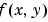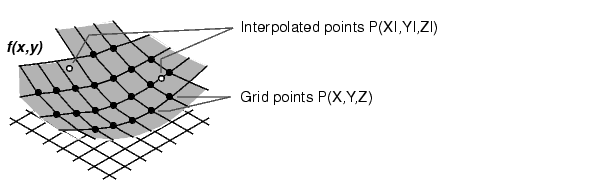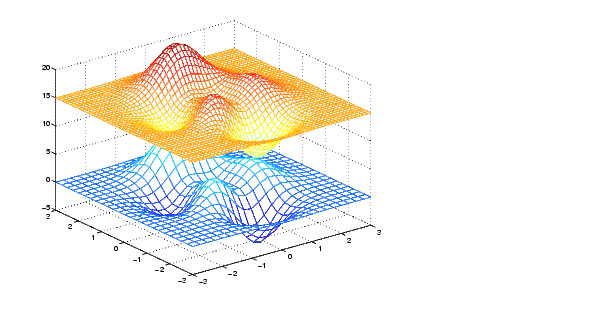MATLAB Function Referenceinterp2

Two-dimensional data interpolation (table lookup)

Syntax

• ```ZI = interp2(X,Y,Z,XI,YI)
ZI = interp2(Z,XI,YI)
ZI = interp2(Z,ntimes)
ZI = interp2(X,Y,Z,XI,YI,method)
ZI = interp2(...,method, extrapval)
```

Description

```ZI = interp2(X,Y,Z,XI,YI) ``` returns matrix `ZI` containing elements corresponding to the elements of `XI` and `YI` and determined by interpolation within the two-dimensional function specified by matrices `X`, `Y`, and `Z`. `X` and `Y` must be monotonic, and have the same format ("plaid") as if they were produced by `meshgrid`. Matrices `X` and `Y` specify the points at which the data `Z` is given. Out of range values are returned as `NaNs`.

`XI` and `YI` can be matrices, in which case `interp2` returns the values of `Z` corresponding to the points `(XI(i,j),YI(i,j))`. Alternatively, you can pass in the row and column vectors `xi` and `yi`, respectively. In this case, `interp2` interprets these vectors as if you issued the command `meshgrid(xi,yi)`.

```ZI = interp2(Z,XI,YI) ``` assumes that `X = 1:n` and` Y = 1:m`, where `[m,n] = size(Z)`.

```ZI = interp2(Z,ntimes) ``` expands `Z` by interleaving interpolates between every element, working recursively for `ntimes`. `interp2(Z)` is the same as `interp2(Z,1)`.

```ZI = interp2(X,Y,Z,XI,YI,method) ``` specifies an alternative interpolation method:

 `'nearest'` Nearest neighbor interpolation `'linear'` Bilinear interpolation (default) `'spline'` Cubic spline interpolation `'cubic'` Bicubuc interpolation

All interpolation methods require that `X` and `Y` be monotonic, and have the same format ("plaid") as if they were produced by `meshgrid`. If you provide two monotonic vectors, `interp2` changes them to a plaid internally. Variable spacing is handled by mapping the given values in `X`, `Y`, `XI`, and `YI` to an equally spaced domain before interpolating. For faster interpolation when `X` and `Y` are equally spaced and monotonic, use the methods `'*linear'`, `'*cubic'`, `'*spline'`, or `'*nearest'`.

```ZI = interp2(...,method, extrapval) ``` specifies a method and a scalar value for `ZI` outside of the domain created by `X` and `Y`. Thus, `ZI` equals `extrapval` for any value of `YI` or `XI` that is not spanned by `Y` or `X` respectively. A method must be specified to use `extrapval`. The default method is `'linear'`.

Remarks

The `interp2` command interpolates between data points. It finds values of a two-dimensional functionunderlying the data at intermediate points.Interpolation is the same operation as table lookup. Described in table lookup terms, the table is `tab` `=` `[NaN,Y;` `X,Z]` and `interp2` looks up the elements of `XI` in `X`, `YI` in `Y`, and, based upon their location, returns values `ZI` interpolated within the elements of `Z`.

Examples

Example 1. Interpolate the `peaks` function over a finer grid.

• ```[X,Y] = meshgrid(-3:.25:3);
Z = peaks(X,Y);
[XI,YI] = meshgrid(-3:.125:3);
ZI = interp2(X,Y,Z,XI,YI);
mesh(X,Y,Z), hold, mesh(XI,YI,ZI+15)
hold off
axis([-3 3 -3 3 -5 20])```

Example 2. Given this set of employee data,

• ```years = 1950:10:1990;
service = 10:10:30;
wage = [150.697 199.592 187.625
179.323 195.072 250.287
203.212 179.092 322.767
226.505 153.706 426.730
249.633 120.281 598.243];
```

it is possible to interpolate to find the wage earned in 1975 by an employee with 15 years' service:

• ```w = interp2(service,years,wage,15,1975)
w =
190.6287
```

See Also

`griddata`, `interp1`, `interp3`, `interpn`, `meshgrid`

© 1994-2005 The MathWorks, Inc.